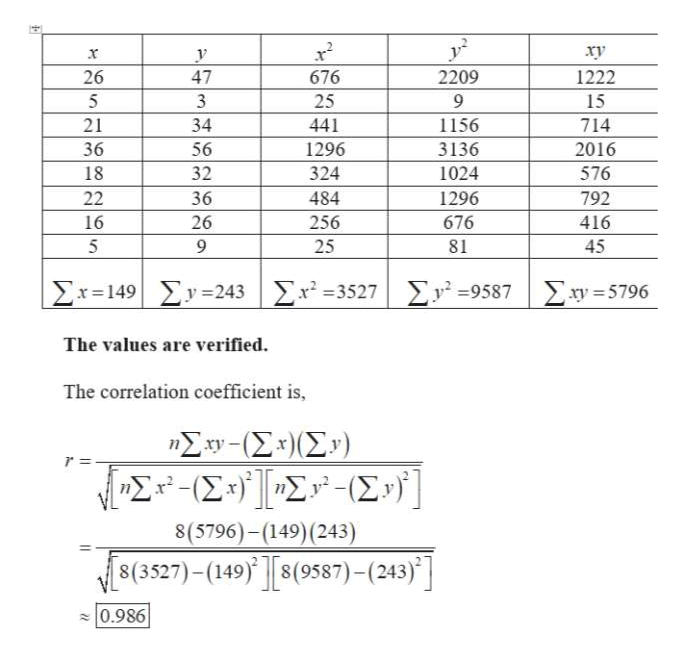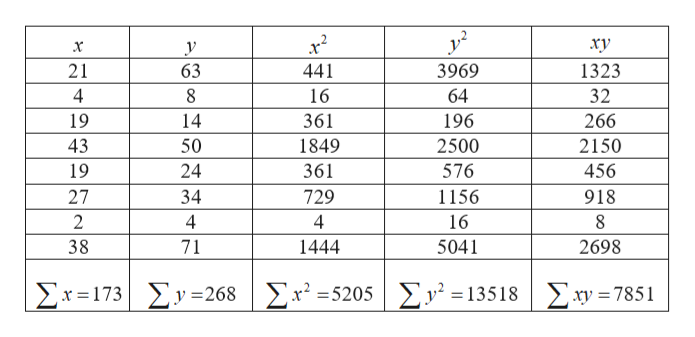# Fuming because you are stuck in traffic? Roadway congestion is a costly item, both in time wasted and fuel wasted. Let x represent the average annual hours per person spent in traffic delays and let y represent the average annual gallons of fuel wasted per person in traffic delays. A random sample of eight cities showed the following data.x (hr)26521361822165y (gal)47334563236269(a) Draw a scatter diagram for the data. Flash Player version 10 or higher is required for this question. You can get Flash Player free from Adobe's website. Verify that Σx = 149, Σx2 = 3527, Σy = 243, Σy2 = 9587, and Σxy = 5796.Compute r.The data in part (a) represent average annual hours lost per person and average annual gallons of fuel wasted per person in traffic delays. Suppose that instead of using average data for different cities, you selected one person at random from each city and measured the annual number of hours lost x for that person and the annual gallons of fuel wasted y for the same person.x (hr)21419431927238y (gal)63814502434471(b) Compute x and y for both sets of data pairs and compare the averages. xyData 1  Data 2  Compute the sample standard deviations sx and sy for both sets of data pairs and compare the standard deviations. sxsyData 1  Data 2  In which set are the standard deviations for x and y larger?The standard deviations for x and y are larger for the first set of data.The standard deviations for x and y are larger for the second set of data.  The standard deviations for x and y are the same for both sets of data.Look at the defining formula for r. Why do smaller standard deviations sx and sy tend to increase the value of r?Dividing by smaller numbers results in a smaller value.Multiplying by smaller numbers results in a larger value.Multiplying by smaller numbers results in a smaller value.Dividing by smaller numbers results in a larger value.(c) Make a scatter diagram for the second set of data pairs. Flash Player version 10 or higher is required for this question. You can get Flash Player free from Adobe's website. Verify that Σx = 173, Σx2 = 5205, Σy = 268, Σy2 = 13,518, and Σxy = 7851.Compute r. (d) Compare r from part (a) with r from part (b). Do the data for averages have a higher correlation coefficient than the data for individual measurements?No, the data for averages do not have a higher correlation coefficient than the data for individual measurements.Yes, the data for averages have a higher correlation coefficient than the data for individual measurements.    List some reasons why you think hours lost per individual and fuel wasted per individual might vary more than the same quantities averaged over all the people in a city.

Question
133 views

Fuming because you are stuck in traffic? Roadway congestion is a costly item, both in time wasted and fuel wasted. Let x represent the average annual hours per person spent in traffic delays and let y represent the average annual gallons of fuel wasted per person in traffic delays. A random sample of eight cities showed the following data.

 x (hr) 26 5 21 36 18 22 16 5 y (gal) 47 3 34 56 32 36 26 9
(a) Draw a scatter diagram for the data.

Flash Player version 10 or higher is required for this question.
You can get Flash Player free from Adobe's website.

Verify that Σx = 149, Σx2 = 3527, Σy = 243, Σy2 = 9587, and Σxy = 5796.

Compute r.

The data in part (a) represent average annual hours lost per person and average annual gallons of fuel wasted per person in traffic delays. Suppose that instead of using average data for different cities, you selected one person at random from each city and measured the annual number of hours lost x for that person and the annual gallons of fuel wasted y for the same person.

 x (hr) 21 4 19 43 19 27 2 38 y (gal) 63 8 14 50 24 34 4 71
(b) Compute x and y for both sets of data pairs and compare the averages.
 x y Data 1 Data 2

Compute the sample standard deviations sx and sy for both sets of data pairs and compare the standard deviations.
 sx sy Data 1 Data 2
In which set are the standard deviations for x and y larger?
The standard deviations for x and y are larger for the first set of data.
The standard deviations for x and y are larger for the second set of data.  The standard deviations for x and y are the same for both sets of data.

Look at the defining formula for r. Why do smaller standard deviations sx and sy tend to increase the value of r?
Dividing by smaller numbers results in a smaller value.
Multiplying by smaller numbers results in a larger value.
Multiplying by smaller numbers results in a smaller value.
Dividing by smaller numbers results in a larger value.

(c) Make a scatter diagram for the second set of data pairs.

Flash Player version 10 or higher is required for this question.
You can get Flash Player free from Adobe's website.

Verify that Σx = 173, Σx2 = 5205, Σy = 268, Σy2 = 13,518, and Σxy = 7851.

Compute r.

(d) Compare r from part (a) with r from part (b). Do the data for averages have a higher correlation coefficient than the data for individual measurements?
No, the data for averages do not have a higher correlation coefficient than the data for individual measurements.
Yes, the data for averages have a higher correlation coefficient than the data for individual measurements.

List some reasons why you think hours lost per individual and fuel wasted per individual might vary more than the same quantities averaged over all the people in a city.
check_circle

Step 1

(a)

The scatter diagram for the provided data can be plotted using Excel.

The steps are given below:

1. Enter the data into Excel sheet.
2. Select the data and click on ‘Insert’ in the tool bar.
3. In the charts, select the scatter plot.
4. Click on ‘Design’ in the toolbar and ‘Add chart element’. Name the axis titles and chart title.

The scatterplot is shown below:help_outlineImage TranscriptioncloseE A 1 x Scatter plot 26 47 3 5 3 60 4 21 34 50 5 36 56 6 18 32 40 7 22 36 30 8 16 26 9 9 20 10 10 11 12 40 10 20 25 30 13 14 15 From the above plot, it can be observed that there is a strong positive correlation between x and y un n fullscreen
Step 2

The calculations are shown below:help_outlineImage Transcriptionclosexy 26 47 676 2209 1222 5 25 9 15 21 34 441 1156 714 36 56 1296 3136 2016 18 32 324 1024 576 22 36 484 1296 792 16 26 256 676 416 5 25 81 45 9 Σ149| Σν-243 | Σ-3527|Σ-587 Σν-5796 The values are verified The correlation coefficient is, ηΣw-(Σ (Σ ) VΣ-ΣΙΣΣν] 8(5796)-(149)(243) [8(3527)-(149)8(9587)-(243) 0.986 fullscreen
Step 3

Consider the second data set and the ...help_outlineImage Transcriptionclosexy 1323 21 63 441 3969 8 16 32 64 19 14 361 266 196 2150 43 50 2500 1849 19 24 361 576 456 27 34 729 918 1156 2 4 4 16 71 1444 38 5041 2698 x =173 Σν-268 y213518 Ety 7851 .r2 5205 fullscreen

### Want to see the full answer?

See Solution

#### Want to see this answer and more?

Solutions are written by subject experts who are available 24/7. Questions are typically answered within 1 hour.*

See Solution
*Response times may vary by subject and question.
Tagged in

### Other